PSAT Math : How to find the domain of a function

Example Questions

2 Next →

Example Question #4 : Algebraic Functions

Find the domain of the function: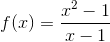All real numbers

All real numbers except for –2

All real numbers except for 1

0

All real numbers except for 1

Explanation:

If a value of x makes the denominator of a equation zero, that value is not part of the domain. This is true, even here where the denominator can be "cancelled" by factoring the numerator into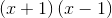and then cancelling the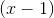from the numerator and the denominator.

This new expression,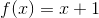is the equation of the function, but it will have a hole at the point where the denominator originally would have been zero. Thus, this graph will look like the line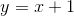with a hole where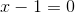, which is.

Thus the domain of the function is allvalues such that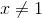2 Next →